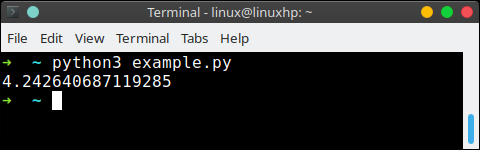If you want to reuse code, you can use a function.

This prevents you from writing the same thing over and over again.

A function has a unique distinct name in the program. Once you call a function it will execute one or more lines of codes, which we will call a code block.

Related Course:

## Function example

For example, we could have the Pythagoras function.

In case your math is a little rusty, a^2 + b^2 = c^2. Thus, c = sqrt( a^2 + b^2). In code we could write that as:

We call the function with parameters `a=3` and `b=3` on the last line.

A function can be called several times with varying parameters. There is no limit to the number of function calls.

The def keyword tells Python we define a function. Always use four spaces to indent the code block, using another number of spaces will throw a syntax error.## Return value

It is also possible to store the output of a function in a variable. To do so, we use the keyword return.

The function pythagoras is called with a=3 and b=3. The program execution continues in the function pythagoras.

The output of math.sqrt(aa + bb) is stored in the function variable value. This is returned and the output is stored in the variable result. Finally, we print variable result to the screen.